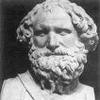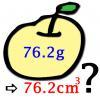#### You may also likeA 1 metre cube has one face on the ground and one face against a wall. A 4 metre ladder leans against the wall and just touches the cube. How high is the top of the ladder above the ground?### Archimedes and Numerical Roots

The problem is how did Archimedes calculate the lengths of the sides of the polygons which needed him to be able to calculate square roots?### More or Less?

Are these estimates of physical quantities accurate?

# Big and Small Numbers in Physics

##### Age 14 to 16Challenge Level

Note that some of these questions involve making estimations and assumptions so these answers are not definitive!

1. Required extra data:
Typical height a person can raise their centre of mass through when jumping on the Earth $h \approx 0.5 \textrm{ m}$.

$\therefore$ under a gravitational field of one-sixth of the strength height reached $h \approx 3.0 \textrm{ m}$

2. Required extra data: None

$\rho_{Pb} = 11.35\ \textrm{g cm}^{-3} \Rightarrow 1 \textrm{ cm}^3$ of lead has mass $m = 11.35\ \textrm{g}$

Mass of one lead atom $m_{A:Pb} = 3.44 \times 10^{-22}\ \textrm{g}$

$\therefore$ number of atoms in $1 \textrm{ cm}^3$

$n = \frac{m}{m_{A:Pb}} = \frac{11.35}{3.44 \times 10^{-22}} = 3.30 \times 10^{22}$

3. Required extra data:
$1$ year = $365.25$ days

$1$ year is one full rotation around Sun, therefore period of orbit in seconds is:
$$T = 365.25 \times 24 \times 60 \times 60 = 31\ 557\ 600\ \textrm{s}$$
The angular velocity of the Earth's orbit is therefore:
$$\omega = \frac{2\pi}{T} = \frac{2\pi}{31\ 557\ 600} = 1.99 \times 10^{-7}\ \textrm{rad s}^{-1}$$
The speed of the Earth relative to the Sun is therefore:
$$v = \omega R = 1.99 \times 10^{-7} \times 149\ 598\ 000\ 000 = 29\ 800\ \textrm{m s}^{-1}$$

4. Required extra data:
Acceleration due to gravity $g = 9.81\ \textrm{m s}^{-2}$

Assuming constant acceleration (i.e. ignoring drag effects), $u = 0 \textrm{ m s}^{-1};\ s = 800\textrm{ m};\ a =g$
$$v^2 = u^2 + 2as \Rightarrow v^2 = 0 + 2(g)(800) \Rightarrow v = 40 \sqrt{g} = 125 \textrm{ m s}^{-1}$$
Note air-resistance is likely to cause the ball to tend towards a terminal velocity below this, typically around $90 \textrm{ m s}^{-1}$

5. Required extra data:
Density of fuel (assume petrol) $\rho_{fuel} \approx 700\ \textrm{kg m}^{-3}$
Typical diameter of fuel tank $D = 4\ \textrm{m}$
Typical length of fuel tank $\ell = 15\ \textrm{m}$

Volume of fuel tank
$$V = \pi \left(\frac{D}{2}\right)^2 \ell = \frac{\pi \times 4^2 \times 15}{4} = 60 \pi\ \textrm{m}^3$$
$\therefore$ weight of fuel in tank
$$W = V \rho_{fuel} = 60 \pi \times 700 = 132\ 000\ \textrm{kg}$$

This seems to be an overestimate as typical values for capacity of a petrol tanker are between 20000 and 40000 litres. What values might be more sensible for the diameter and length?

6. Required extra data:
Hydrochloric acid is a strong acid (complete dissociation) $\Rightarrow \textrm{pH} = -\log_{10} [\textrm{H}^+]$
Avogadro's constant $N_A = 6.022 \times 10^{23}\ \textrm{mol}^{-1}$

$$\textrm{pH} = 1.0 \Rightarrow [\textrm{H}^+] = 10^{-1.0} = 0.1 \textrm{ mol dm}^{-3}$$
$$\therefore 0.1 \textrm{ mol in } 1 \textrm{ dm}^3 (1\ \ell) \textrm{ which is } 0.1N_A = 6.022 \times 10^{22}\textrm{ hydrogen ions}$$
$$\therefore \textrm{ total sum of positive charges } = 6.022 \times 10^{22} \times 1.6 \times 10^{-19} = 9635 \textrm{ C}$$

7. Required extra data:
Relative molecular mass of water $m_{R} = 18.02\ \textrm{u}$
Avogadro's constant $N_A = 6.022 \times 10^{23}\ \textrm{mol}^{-1}$

1 mole of water has a mass $m = 18.02\ \textrm{g}$
There are $N_A$ particles in 1 mole of a substance $\therefore$ one molecule of water has mass
$$m = \frac{18.02}{6.022 \times 10^{23}} = 2.992 \times 10^{-23}\ \textrm{g}$$

8. Required extra data:
Answer to Q7. (mass of one molecule of water) $m = 2.992 \times 10^{-23}\ \textrm{g}$
Typical volume of an ice cube - assume dimensions $2\ \textrm{cm} \times 2\ \textrm{cm} \times 3\ \textrm{cm} \Rightarrow V = 12\ \textrm{cm}^3$
Density of ice $\rho_{ice} = 0.917\ \textrm{g cm}^{-3}$

Mass of ice cube
$$M = \rho_{ice}V = 0.917 \times 12 = 11.004\ \textrm{g}$$
$\therefore$ number of molecules in one ice cube is
$$n \approx \frac{11.004}{2.992 \times 10^{-23}} = 3.7 \times 10^{23}$$

9. Required extra data:
Speed of electromagnetic radiation (in free space) $c = 299\ 792\ 458\ \textrm{m s}^{-1}$

$$T = 13.4\ \textrm{billion years} = 13.4 \times 10^9 \times 365.25 \times 24 \times 60 \times 60 = 4.229 \times 10^{17}\ \textrm{s}$$
$$\therefore d = cT = 299\ 792\ 458 \times 4.229 \times 10^{17} = 1.27 \times 10^{26}\ \textrm{m}$$

10. Required extra data:
Mass of Earth $M_E = 5.9742 \times 10^{24}\ \textrm{kg}$
Speed of electromagnetic radiation (in free space) $c = 299\ 792\ 458\ \textrm{m s}^{-1}$

Einstein's famous formula relating energy to mass is:
$$E = mc^2 \therefore E_{Earth} = M_E c^2 = 5.9742 \times 10^{24}\times 299\ 792\ 458^2 = 5.369 \times 10^{41}\ \textrm{J}$$